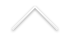Probability Seminar ''On a Dyson-fractional Brownian motion'' November 27, from 3:30 p.m. to 4:30 p.m. (Rio de Janeiro local time)

Local: Transmissão Online

Clique AQUI para acessar a transmissão.

Speaker: Victor Pérez-Abreu (Guanajuato, Mexico)

Abstract: This talk will present an overview of the behavior of the eigenvalues of the fractional Brownian matrix motion and other related matrix processes. We emphasize on a possible extension of the Dyson-Brownian motion, namely the dynamics of the eigenvalues processes and their non-colliding property, the limit of the associated empirical process, as well as the free Brownian motion and the non commutative fractional Brownian motion.

More complete information about the seminars can be found at DME.

Sincerely,

Organizers: Giulio Iacobelli e Maria Eulalia Vares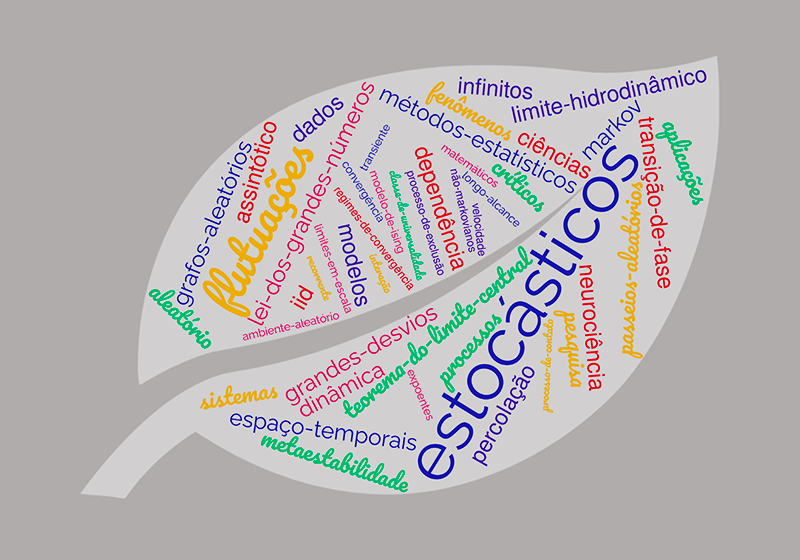Probability Seminar "Stein’s method and asymptotic independence" November 6, from 3:30 p.m. to 4:30 p.m. (Rio de Janeiro local time)

Speaker: Ciprian Tudor (Université de Lille)
Abstract: If Y is a random vector in R^d we denote by P_Y its probability distribution. Consider a random variable X and a d-dimensional random vector Y. We develop a multidimensional variant of the Stein-Malliavin calculus which allows to measure the Wasserstein distance between the law P_(X, Y) and the probability distribution P_Z x P_Y, where Z is a Gaussian random variable. That is, we give estimates, in terms of the Malliavin operators, for the distance between the law of the random vector  (X, Y) and the law of the vector (Z,Y), where Z is Gaussian and independent of Y.

Then we focus on the particular case of random vectors in Wiener chaos and we give an asymptotic version of this result. In this situation, this variant of the Stein-Malliavin calculus has strong and unexpected consequences.

More complete information about the seminars can be found at DME.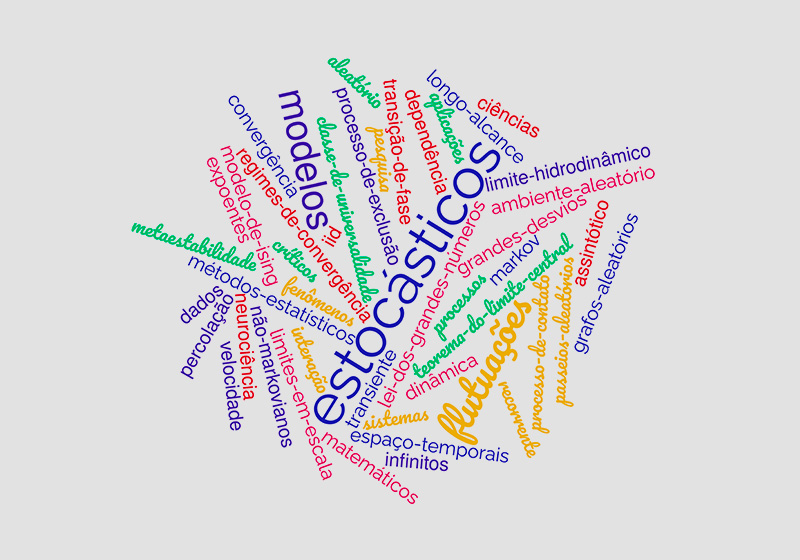De 4 a 8 de dezembro de 2023 ocorrerá o minicurso The cutoff phenomenon for stochastic Langevin equations, ministrado pelo Professor Michael Högele, da Universidad de Los Andes, Bogotá.

Para saber mais, clique AQUI.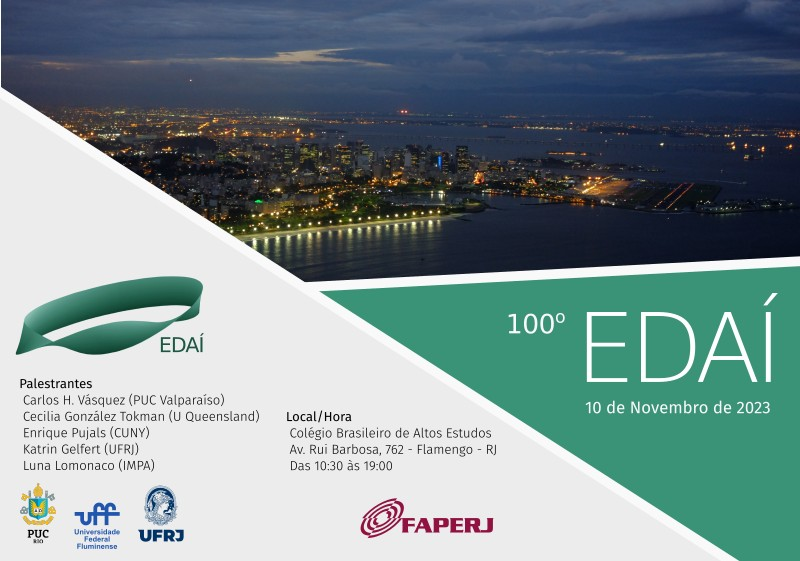100º EDAÍ, 10/11 no CBAE em Flamengo

Local: Colégio Brasileiro de Altos Estudos, Av. Rui Barbosa, 762 - Flamengo

Data e hora: 10 de novembro de 2023, das 10:30 às 18:30

10h30 – 11h30: Katrin Gelfert (UFRJ)

Ergodic recycling of heterodimensional cycles

We define the concept of heterodimensional cycles between hyperbolic ergodic measures of different indices. We study what impact the existence of such a cycle has on the topological properties of the space of invariant measures and present some examples. This is joint work with L. Díaz and Ch. Bonatti.

11h45 - 12h45: Carlos H. Vásquez (PUC Valparaíso)

Measures of maximal entropy for partially hyperbolic diffeomorphisms.

A central problem in dynamics is being able to determine the existence of measures that capture relevant dynamic information. One of these measures is the one that maximizes entropy. In this talk, we will explore the problem of establishing the existence and uniqueness of this measure in partially hyperbolic systems.

14h30 - 15h30: Luna Lomonaco (IMPA)

The Mandelbrot set and its Satellite copies

For a polynomial on the Riemann sphere, infinity is a (super) attracting fixed point, and the filled Julia set is the set of points with bounded orbit. Consider the quadratic family P_c(z)=z^2+c. The Mandelbrot set M is the set of parameters c such that the filled Julia set of P_c is connected.

Computer experiments quickly reveal the existence of small homeomorphic copies of M inside itself; the existence of such copies was proved by Douady and Hubbard. Each little copy is either primitive (with a cusp on the boundary of its main cardioid region) or a satellite (without a cusp). Lyubich proved that the primitive copies of M satisfy a stronger regularity condition: they are quasiconformally homeomorphic to M. The satellite copies are not quasiconformally homeomorphic to M (as we cannot straighten a cusp quasiconformally), but are they mutually quasiconformally homeomorphic? In joint work with C. Petersen we prove that the answer is negative in general, but positive in the case the satellite copies have rotation number with same denominator (this last part is work in progress).

15h40 - 16h40: Cecilia González Tokman (University of Queensland - UQ)

Lyapunov–Oseledets spectrum for transfer operator cocycles under perturbations

In recent years, the study of transfer operators has been combined with multiplicative ergodic theory to shed light on ergodic-theoretic properties of random dynamical systems. The so-called Lyapunov– Oseledets spectrum associated to the transfer operator cocycle contains fundamental information about invariant measures, exponential decay rates and coherent structures which characterize dominant global transport features of the system. While the scope of this framework is broad, it is challenging to identify and approximate this spectrum. In this talk, we present examples of maps where the Lyapunov– Oseledets spectrum can be understood and analyzed under perturbations. This talk is based on joint work with Anthony Quas.

17h10 – 18h10: Enrique Pujals (City University of New York - CUNY)

From zero to positive entropy

We will discuss the mathematical processes by which a system evolves from one whose recurrent set is finite towards another one exhibiting chaotic behavior as parameters governing the behavior of the system are varied. In that direction, we will present a tentative global framework toward describing a large class of two-dimensional dynamics (that includes the Henon family), inspired partially by the developments in the one-dimensional theory of interval maps. More precisely, we present a class of intermediate smooth dynamics between one and higher dimensions where it is possible to describe the transition from zero to positive entropy.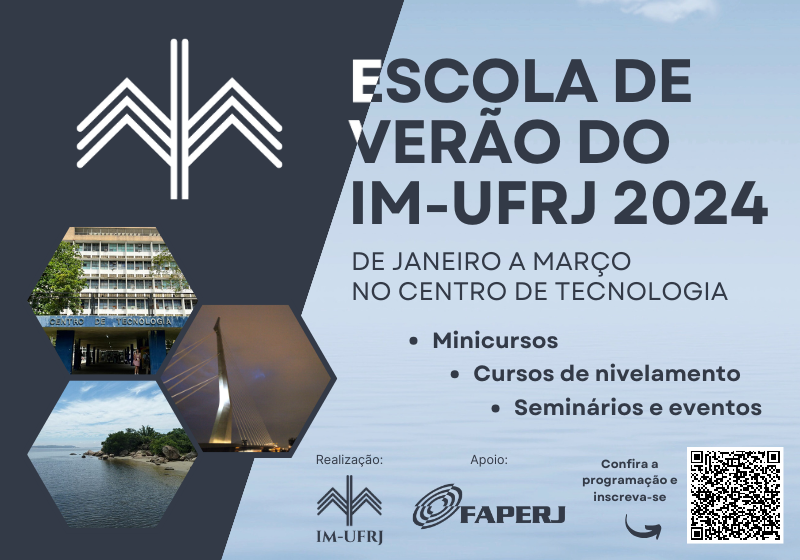De janeiro a março de 2024 acontecerá o Escola de verão - IM-UFRJ

Minicursos Confirmardos:

Introdução na teoria de controle ótimo e equações de Hamilton - Jacobi - Erwin Topp (IM-UFRJ)

Um breve passeio pelas interseções entre música, matemática e estatistica - Hugo Tremonte de Carvalho (IM-UFRJ)

Introdução às matemágicas - Bernardo Nunes Borges de Lima (ICEX - UFMG)

Superficies minimas - Haimer Alexander Trejos Serna (UERJ)

Activated Random Walks on Z°- Leonardo Trivellato Rolla (IME-USP)

Confira a Programação completa AQUI.